# Reducing Variation During Design

Design offers the first and best opportunity for reducing variation.  To illustrate the strategies and tools required, consider the task of designing a new pump.  Suppose we are faced with the task of designing a pump capable of delivering solution at a constant rate of 10 ml/min.  Customer usage requires that the flow rate remains between 9 and 11 ml/min.  The first step is to develop a general design concept.  Suppose, we decide to use a piston to push the solution.  This concept requires a motor to drive the piston and two valves to control the direction of the flow.

Next, we need to determine all the specifics such as “How far should the piston travel?” and “How fast should the motor turn?”  To accomplish this task, we need to make a list of all the factors affecting flow rate.  Three obvious factors are the piston radius (R), the stroke length (L), and the motor speed (S).  Another possible factor is the amount of backflow through the valves (B).  Other factors might also be considered such as the solution viscosity.  But, for purpose of example, we will limit ourselves to the first four factors.  They are shown to the left in Figure 1.  They will be referred to as the input variables.  Flow rate will be referred to as the output variable.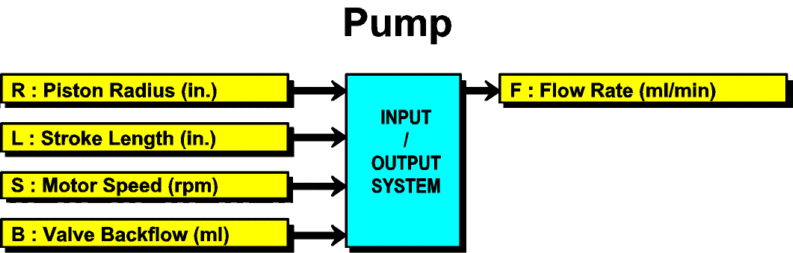Figure 1: Factors Affecting Flow Rate

It is the output for which we desire to reduce the variation.  This is accomplished through the establishments of requirements for the inputs.  This requires knowledge of how the inputs behave and how the inputs affect the output.  Based on this information we can predict how the outputs will behave.

VARIATION TRANSMISSION ANALYSIS

We will start with how the inputs affect the output.  For our pump, it is possible to derive the following equation for flow rate: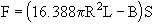(Eq. 1)

The term πR2L is the volume of a cylinder of radius R and length L.  This volume of solution is displaced each piston stroke.  This is multiplied by 16.388 to convert the volume from cubic inches to milliliters.  The backflow per stroke B is then subtracted and the result multiplied by the motor speed S to give the flow rate F in ml/min.  While the equation is readily available in this example, in many cases screening experiments and response surface studies are required to identify the key input variables and to obtain an empirical equation.

Based on Equation 1, the flow rate average and standard deviation can be determined as a function of the averages and standard deviations of the inputs.  Let μF and σF denote the average and standard deviation of flow rate.  Then the resulting formulas are: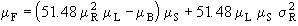(Eq. 2)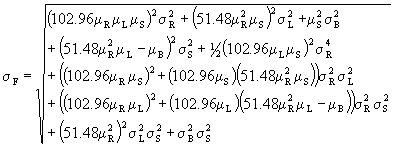(Eq. 3)

These equations were obtained using the methods detailed in Taylor (1991), Cox (1986) and Evans (1975).  These approaches have a variety of names including statistical tolerance analysis and propagation of errors.  The term variation transmission analysis will be used here to collectively refer to the different methods of predicting the behavior of the output.

SPECIFYING TOLERANCES

The last thing required is information on how the inputs behave.  This might require calling the motor supplier to determine the capability of their process in controlling motor rpm.  The valve supplier might also have to be contacted to determine the average and standard deviation of valve backflow.  One might also have to call the plant to obtain process capability information on drilling the piston hole.  In many cases, one will have choices as to the components and processes to use.  In this case, one should start with the low-cost components and processes for the initial design.  Changes can be made later if necessary.  Table 1 shows the information collected.

Table 1: Data Collected From Plants and Suppliers

To ensure the design is manufacturable, initial requirements will be established based on these low-cost tolerances.  These requirements are shown in Table 2. These requirements are specified as process tolerances.  Process tolerances specify a minimum and maximum value for the average and a maximum standard deviation.  They specify requirements for the processes producing the components rather than for the individual components themselves.  This facilitates the communication of design requirements to the plants and prevents differences in interpretation.  The maximum standard deviations were set equal to the standard deviations in Table 1 while the tolerances for the averages were set equal to ± 1.5 standard deviations.  In addition, targets must be selected for piston radius (R), stroke length (L), and motor speed (S).  Initial values of R = 0.1″ and L = 0.5″ were selected based on judgment.  Equation 1 was then used to calculate the required motor speed.

Table 2: Process Tolerances for Inputs

For the process tolerances given in Table 2, Equations 2 and 3 were used to determine the resulting process tolerance for flow rate (F).  The results are shown in Figure 2.  In addition, worst-case values of Cp and Cpk are given along with the six sigma product tolerance.  The six sigma product tolerance represents an interval containing all but 3.4 units per million.  We want the six sigma product tolerance to fit between the specification limits which is equivalent to Cpk exceeding 1.5.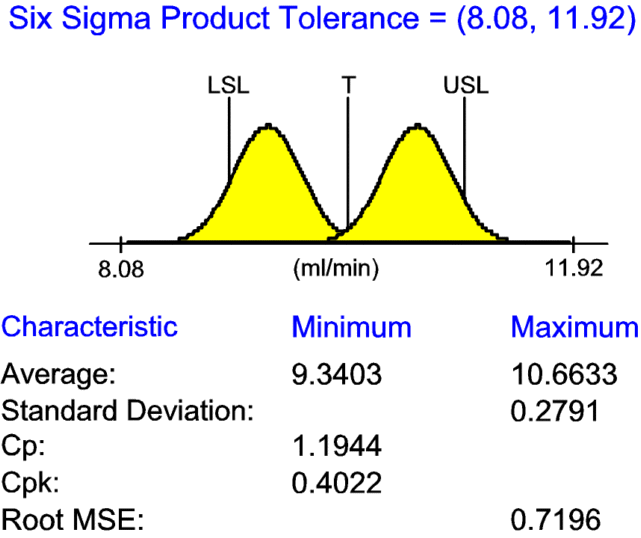Figure 2: Flow Rate Variation Based on Table 2

ROBUST DESIGN THROUGH SELECTION OF OPTIMAL TARGETS

One way of reducing the flow rate variation is through robustness.  Robustness is obtained by designing the pump to be less sensitive to the variation of the inputs.  Therefore, the inputs continue to vary, but less of this variation is transmitted to the output.  As a result, the output varies less. Robust design is accomplished through the setting of the inputs’ targets.  Equation 3 clearly indicates that the targets affect the variation.  Since it is generally less costly to adjust targets than tighten tolerances, building in robustness is the preferred method of reducing variation.

There are many different combinations of the inputs’ targets that result in a flow rate of 10 ml/min.  Lengthening the stroke length can be offset by decreasing the piston radius or reducing the motor speed.  However, as Equation 3 demonstrates, the inputs’ targets also affect the variation.  Of those targets resulting in an average flow rate of 10 ml/min, the set of targets that minimizes the flow rate variation is desired.

A robust pump design can be obtained by determining the targets of the inputs maximizing the minimum Cpk.  For the pump, the optimal set of targets for the inputs are:

R = 0.1735″ L = 0.4125″ S = 16.96 rpm

The resulting flow rate variation is shown in Figure 3.  The new predicted product tolerance is much closer to the spec. limits and the minimum Cpk is closer to 1.5.  However, further improvements are still required.  At this point, further improvements can only be made through tightening tolerances.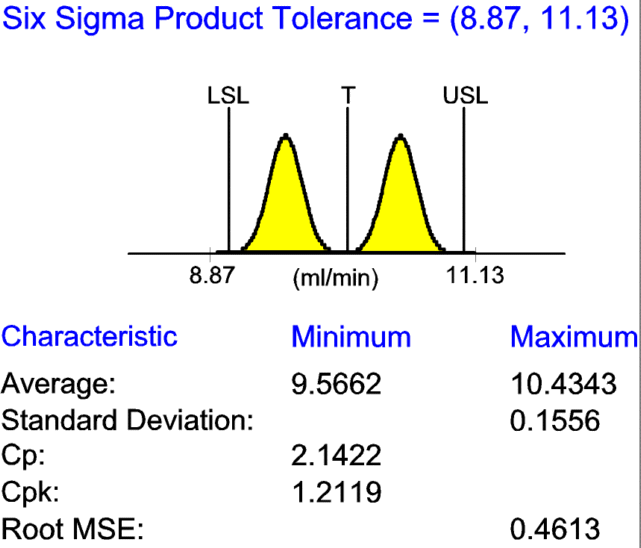Figure 3: Flow Rate Variation Using Optimal Targets

TIGHTENING TOLERANCES

Which tolerance or tolerances should we tighten and by how much?  To start to answer this question, it is useful to investigate the effects of tightening the different tolerances.  Table 3 shows the effect of reducing the tolerance to zero for each of the inputs.  Tightening the motor speed and backflow tolerances have the largest effects.

Table 3: Flow Rate Process Tolerances After Tightening Tolerances

Which tolerance one decides to tighten also depends on the costs involved.  Tightening the motor speed tolerance requires the substitution of a \$20 motor for the current \$5 motor.  Tightening the valve tolerance requires the use of a \$2 valve instead of the current \$1 valve. I n this case the best option is to use the \$2 valve.  This valve has an average backflow of 0.05 ml with a standard deviation of 0.0025 ml.  A tolerance for the average of 0.05 ± 0.00375 ml and maximum standard deviation of 0.0025 ml will be used for its process tolerance.

The current targets were selected to be optimal for the low-cost tolerances.  After making a change in the tolerances, the targets should be reoptimized.  The new set of targets are:

R = 0.1520″ L = 0.4236″ S = 22.04 rpm

The resulting flow rate variation is shown in Figure 4. The predicted product tolerance fits inside the spec. limits and the minimum Cpkexceeds 1.5. The pump design now meets the six sigma criteria.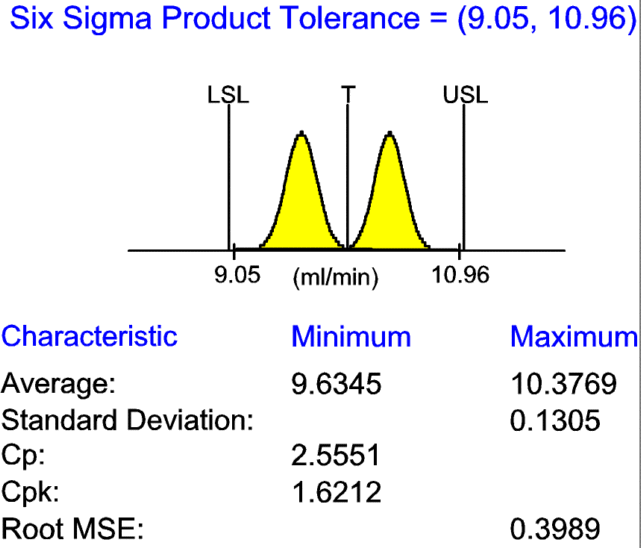Figure 4: Flow Rate Variation Using The \$2 Valve

SUMMARY

The above example illustrates the following strategies:

1. Knowledge of how the inputs behave and how the inputs affect the output is the key to reducing variation during design. – Otherwise trial and error is necessary.
2. The use of robust design by considering variation while setting targets. – Instead, variation reduction is commonly accomplished through tightening of tolerances which drives up costs.
3. Obtaining manufacturable products by starting with low-cost tolerances and selectively tightening tolerances based on specific component and process changes. – Instead, tight tolerances are commonly specified for all the inputs to ensure design performance. Manufacturability is only considered late in the design.
4. Using process tolerances to clarify the designer’s intent when setting tolerances. – Instead, product tolerances are commonly specified based on both statistical tolerancing and worst-case tolerance without clear guidance on how to interpret them.

Implementing these strategies requires that variation begin to be addressed in the earliest stages of design where changes are less costly. Otherwise, the opportunity to design in robustness and to base the design on manufacturing capability is lost. If the variation is not addressed early in design, variation must be reduced later by tightening tolerances which drives up costs.

REFERENCE LIST

Cox, N. D. (1986). How to Perform Statistical Tolerance Analysis. Milwaukee: ASQC Quality Press.

Evans, D. H. (1975). Statistical Tolerancing:: The State of the Art. Journal of Quality Technology 7 (1): 1-12.

Taylor, W. A. (1991). Optimization and Variation Reduction in Quality. New York: McGraw-Hill.

Appeared in SOCE News, The Society of Concurrent Engineering, Spring 1996, p. 1-6

Presented at MD&M West, February 6-8, 1996

Presented at MD&M East, June 4-6, 1996